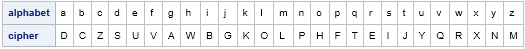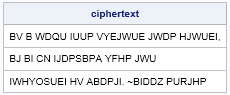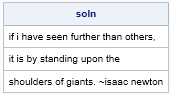Today is my fourth blog-iversary: the anniversary of my first blog post in 2010. To celebrate, I am going to write a series of fun posts based on The Code Book by Simon Singh, a fascinating account of the history of cryptography from ancient times until the present. While reading the book, I was struck by the number of statistical concepts that are involved in cryptography.

I will blog about cryptography once or twice a month (Tag: Ciphers). My focus will be statistical ideas in classical cryptography, from ancient Rome through World War II. Topics will include substitution ciphers, frequency analysis of letters and pairs of letters, and how to construct and solve recreational puzzles, such the popular Cryptoquote puzzle that appears in many newspapers.

As usual, each article will be accompanied by a SAS program that demonstrates a technique in statistical programming. Even though most of us will never encounter secret messages as part of our professional work, these posts will demonstrate useful techniques for writing statistical programs in SAS/IML language.

But enough talk! Let's get started by defining some basic terms:

• A substitution cipher is a permutation of an alphabet. Each letter of the alphabet is transformed under the permutation into another (not necessarily different) letter, and the ordered set of permuted letters is the cipher. Enciphering is the process of replacing each letter in a text by its counterpart in the cipher. Deciphering is the process of applying the inverse permutation to an enciphered message to recover the original message.
• The message prior to encryption is called the plaintext message. The alphabet in its natural order is the plaintext alphabet. Traditionally, plaintext is written in all lowercase letters.
• The encrypted message is called the ciphertext. Traditionally, ciphertext is written in all uppercase letters.

### Substitution ciphers and keys

The substitution cipher has been used to encipher messages since the time of Julius Caesar. Its simplicity kept it in use until the Renaissance, even though Arab mathematicians developed frequency analysis in the ninth century and the Europeans knew how to break substitution ciphers for long messages in the 1500s. Today the substitution cipher is used mainly to construct recreational puzzles.

A substitution cipher is determined by a key that, in conjunction with some algorithm, transforms a plaintext alphabet into a cipher alphabet. A special case of the substitution cipher is the simple Caesar cipher (or shift cipher), which has only 25 possible keys and is therefore susceptible to a brute force attack (just check all possible shifts). However, there are 26! = 4 x 1026 permutations of 26 letters, so the general substitution cipher is resistant to a naive brute force attack.

Before the modern computer, general substitution ciphers were not used often because the agents would need to remember the complete 26-element permutation, and the process of encipherment by hand was slow and subject to error. However, if you have access to a programming language that includes a random number generator, the key can be any easily remembered seed for the generator.

Here's how a programming language like SAS/IML makes it simple to encipher a message. First, choose a random number seed as the encryption key. Then create a cipher by using the SAMPLE function to sample without replacement ("WOR") from the plaintext alphabet. For example, I might choose 432013 as the secret key because I can remember that my book Simulating Data with SAS was published on April 3, 2013. The following SAS/IML program constructs the cipher:

```proc iml; key = 432013; call randseed(key, 1); /* reset random number stream; initialize with key */ alphabet = "a":"z"; /* plaintext alphabet */ cipher = upcase(sample(alphabet, 26, "WOR") ); /* permutation of alphabet */ print (alphabet//cipher)[r={"alphabet" "cipher"}];```The output shows the cipher alphabet beneath the plaintext alphabet. For the key 432013, the letter 'a' is enciphered as 'D', 'b' goes to 'C', 'c' goes to 'Z', and so on. For this permutation, 'x' is enciphered as 'X', so not every letter is changed by the encipherment.

The program uses a trick that you might not have seen before. The RANDSEED subroutine in SAS/IML has an optional second argument. If the second argument has the value 1, then the random number generator is reset. By calling the RANDSEED subroutine in this way, you can ensure that the encipherment does not depend on the current state of the random number generator.

You can use the TRANSLATE function in Base SAS to replace every element in the plaintext alphabet by the associated letter in the enciphered alphabet. The ROWCAT function in SAS/IML concatenates the 26-element arrays into a single character string with 26 letters. The following statements enciphers one of my favorite quotes by Isaac Newton:

```msg = {"If I have seen further than others, ", "it is by standing upon the ", "shoulders of giants. ~Isaac Newton"}; plaintext = lowcase(msg); ciphertext = translate(plaintext, rowcat(cipher), rowcat(alphabet)); print ciphertext;```The output of the program is an enciphered message that is in the spirit of the Cryptoquote puzzle. Use this technique to construct puzzles for your puzzle-loving friends!

### Modules to encipher and decipher messages

If you and your friend want to exchange messages by using a substitution cipher, you need to agree on a key and an algorithm for constructing a permutation of the alphabet. The following SAS/IML modules use the SAMPLE function in SAS/IML to construct a cipher from the key. The Encipher module applies the permutation to a message, thus encrypting it. The Decipher module applies the inverse permutation, thus decrypting the enciphered text.

```start Encipher(key, msg); call randseed(key, 1); /* reset and initialize random number stream */ alphabet = "a":"z"; cipher = upcase( sample(alphabet, 26, "WOR") ); /* permutation of alphabet */ ciphertext = translate(lowcase(msg), rowcat(cipher), rowcat(alphabet)); return (ciphertext); finish;   start Decipher(key, msg); call randseed(key, 1); /* reset and initialize random number stream */ alphabet = "a":"z"; cipher = upcase( sample(alphabet, 26, "WOR") ); /* permutation of alphabet */ plaintext = translate(upcase(msg), rowcat(alphabet), rowcat(cipher)); return (plaintext); finish;   msg = {"If I have seen further than others, ", "it is by standing upon the ", "shoulders of giants. ~Isaac Newton"}; cryptogram = Encipher(key, msg); soln = Decipher(key, cryptogram); print soln;```Now you and your friends can exchange enciphered messages such as "Want to meet for lunch today at eleven forty-five?" Try it out and let me know what you think. But remember not to say nasty things about your boss: the substitution cipher is not a secure means of communication, as I will demonstrate in a future blog post.

Tags Ciphers
ShareDistinguished Researcher in Computational Statistics

Rick Wicklin, PhD, is a distinguished researcher in computational statistics at SAS and is a principal developer of SAS/IML software. His areas of expertise include computational statistics, simulation, statistical graphics, and modern methods in statistical data analysis. Rick is author of the books Statistical Programming with SAS/IML Software and Simulating Data with SAS.

1.2.